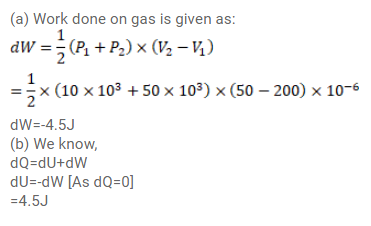# The pressure of a gas changes linearly with volume fromQuestion:

The pressure of a gas changes linearly with volume from $10 \mathrm{kPa}, 200 \mathrm{cc}$ to $50 \mathrm{kPa}, 50 \mathrm{cc}$.

(a) Calculate the work done by the gas.

(b) If no heat is supplied or extracted from the gas, what is the change in the internal energy of the gas?

Solution: